﻿ A Comparative Study on Bayesian Optimization Algorithm for Nutrition Problem

### A Comparative Study on Bayesian Optimization Algorithm for Nutrition Problem

Serpil Gumustekin, Talat Senel, Mehmet Ali CengizOPEN ACCESSPEER-REVIEWED

## A Comparative Study on Bayesian Optimization Algorithm for Nutrition Problem

Serpil Gumustekin1,, Talat Senel1, Mehmet Ali Cengiz1

1Department of Statistics, Faculty of Arts and Science, Ondokuzmayis University, Samsun, Turkey

### Abstract

In this paper, the Bayesian Optimization Algorithm (BOA), which is one of the multivariate EDA algorithms with graphical model, was investigated. Then BOA was applied to the problem of nutrition for breakfast. The results obtained from BOA were compared to Genetic Algorithm and Linear Programming. At the end of the comparisons, for the problem of a recommended diet for breakfast, BOA approach gives more effective results than the other mentioned methods in terms of time and the cost.

### At a glance: Figures

1
Prev Next

• Gumustekin, Serpil, Talat Senel, and Mehmet Ali Cengiz. "A Comparative Study on Bayesian Optimization Algorithm for Nutrition Problem." Journal of Food and Nutrition Research 2.12 (2014): 952-958.
• Gumustekin, S. , Senel, T. , & Cengiz, M. A. (2014). A Comparative Study on Bayesian Optimization Algorithm for Nutrition Problem. Journal of Food and Nutrition Research, 2(12), 952-958.
• Gumustekin, Serpil, Talat Senel, and Mehmet Ali Cengiz. "A Comparative Study on Bayesian Optimization Algorithm for Nutrition Problem." Journal of Food and Nutrition Research 2, no. 12 (2014): 952-958.

 Import into BibTeX Import into EndNote Import into RefMan Import into RefWorks

### 1. Introduction

Nutrition is the foundation of health at every period of life. It is sometimes done consciously or sometimes unconsciously. Scientists have been working on adequate and balanced diets for many years. Nutrition is the supply of food required by organisms and cells to stay alive. In science and human medicine, nutrition is the science or practice of consuming and utilizing foods. Various nutrients are essential to build up and maintain healthy cell tissues, glands and organs in the human body. Without specific nutrients the body is not able to perform many of its functions, be they metabolic, mental, physical or chemical. The nutrients are available only through food which is one of the most essential factors in maintaining and preserving health. The scientific research has revealed that there is a requirement for more than 40 kinds of nutrients for human life. Also, they determined that each of these nutrients must be received daily.

Mathematical programming techniques are widely used in the analysis of nutritional problems. They have been widely studied in recent years, and an extensive summary of the approaches taken can be found in (Sukhatme, 1961); (Edwardson, 1974); (Anderson and Earle, 1983); (Alpaslan, 1996); (Kaldırım and Kose,2006); (Lv,2009); (Sahingöz and Sanlıer, 2011).

An optimization problem is the challenge to ﬁnd the optimal or near optimal solution from a speciﬁed set of feasible solutions using some measure for evaluating each individual solution. The task is to find the solution of the highest quality. The Nutrition Problem was chosen because of its historical significance, instructional value and ease of understanding. It can be easily stated as:

Minimize the cost of food eaten during breakfast and subject to the requirements that the breakfast satisfy a person's nutritional requirements and that not too much of any one food be eaten. Linear programming was developed as a technique by (George B. Dantzig, 1947).

Genetic algorithms are search algorithms based on the mechanics of natural selection and natural genetics. In a GA, two genetic operators, crossover and mutation, are used to simulate the variation. Crossover forms the new population by exchanging some parts between the selected solutions. Mutation slightly modiﬁes some parts of the newly formed solutions to introduce some genetic variation in the new population. The traditional crossover and mutation approach of variation in GAs has been found to be limited for many optimization problems, and therefore, most of the early research in GAs has been focused on the modiﬁcation of these operators to improve GA performance. In recent years, a probabilistic approach to variation has been proposed where crossover and mutation is replaced by two other operators: distribution estimation and sampling. Distribution estimation is to estimate a probability distribution of solutions from a population, and sampling is to sample the distribution to generate a new population. Algorithms using such an approach to variation are called Estimation of Distribution Algorithms (EDAs) (Muhlenbein & Paaβ, 1996; Larranaga & Lozano, 2002). In the literature, EDAs are also called probabilistic model building genetic algorithms (PMBGA) and iterated density estimation algorithms (IDEAs).

Bayesian Optimization Algorithm (BOA) is a specific kind of EDA proposed by (Pelikan et al, 1999) for solving a group of complicated problems efficiently. It combines the idea of using probabilistic models to guide optimization and the methods for learning Bayesian networks. To learn a proper decomposition of the problem, BOA builds a Bayesian network for the set of promising solutions. New candidate solutions are generated by sampling the built network. BOA generates the initial population of strings at random with a uniform distribution over all possible strings. The population is updated for a number of iterations (generations), each consisting of four steps. First, promising solutions are selected from the current population using a GA selection method, such as tournament and truncation selection. Second, a Bayesian network that fits the population of promising solutions is constructed. Third, new candidate solutions are generated by sampling the built Bayesian network. Fourth, the new candidate solutions are incorporated into the original population, replacing some of the old ones or all of them. The above four steps are repeated until some termination criteria are met.

Bayesian network (Pearl, 1998) was used in the proposed Bayesian optimization algorithm to solve the nutrition problem. A Bayesian network is a directed acyclic graph with each node corresponding to one variable, and each variable corresponding to the individual rule by which a nutrition problem will be constructed step by step. The causal relationship between two variables is represented by a directed edge between the two corresponding nodes. The Bayesian optimization algorithm is applied to learn to identify good partial solutions and to complete them by building a Bayesian network of the joint distribution of solutions (Pelikan et al, 1999); (Pelikan and Goldberg, 2000). The conditional probabilities are computed according to an initial set of promising solutions. Subsequently, each new instance for each node is generated by using the corresponding conditional probabilities, until values for all nodes have been generated. If stopping conditions are not met, the conditional probabilities for all nodes in the Bayesian network are updated again using the current set of rule strings. The algorithm thereby tries to explicitly identify and mix promising building blocks.

In our nutrition problem, a linear programming model was constructed using real data to meet the needs of the average daily nutrient requirement for adequate and balanced nutrition with 10 nutrients and 20 foodstuffs for breakfast. Then the model was solved by using Bayesian Optimization Algorithm (BOA), Genetic Algorithm (GA) and Linear Programming (LP). The results were then compared to each other. The results obtained with a Bayesian optimization algorithm show that reduced time and cost savings were achieved.

### 2. Materials and Methods

The first step is to assign variables for the amounts of food that we are looking for and parameters for the data that we know.

2.1. Linear Programming

In mathematical terms, linear programming is a tool to optimize (minimize or maximize) a linear function of a set of decision variables while respecting multiple linear constraints.

The Nutrition Problem can be formulated mathematically as a linear programming problem as shown below.

Sets:

F: Set of foods

N: Set of nutrients

Parameters: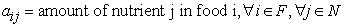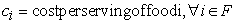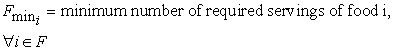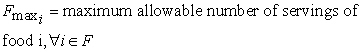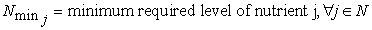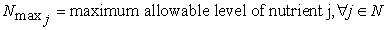Decision Variables: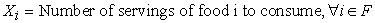Objective Function:

Minimize the total cost of the breakfast.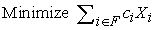(1)

Constraints:

Constraint Set 1: For each nutrient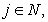at least meet the minimum required level.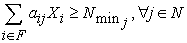(2)

Constraint Set 2: For each nutrientdo not exceed the maximum allowable level.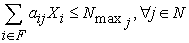(3)

Constraint Set 3: For each food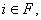select at least the minimum required number of servings.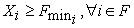(4)

Constraint Set 4: For each fooddo not exceed the maximum allowable number of servings.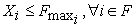(5)

2.1.1. Objective function

We express our decision variables as follows. X1:Bread, X2:Sausage, X3:Wiener, X4:Salami, X5:Milk, X6:White Cheese, X7:Cheddar Cheese, X8: Tulum Cheese, X9:Curd Cheese, X10: Egg, X11: Butter, X12: Margarine, X13: Tomato, X14: Cucumber, X15:Black Olives, X16:Green Olives, X17:Jam, X18:Honey, X19:Sesame Oil, X20:Boiled Grape Juice. The prices corresponding to 1 gram of the nutrients eaten for breakfast are given in the Table 1 and Nutritional composition of foods (100 grams) eaten for breakfast are given in the Table 2.

#### Table 2. Nutritional composition of foods (100 grams) for breakfast

We created objective function with the help of the Eq.1 is as follows.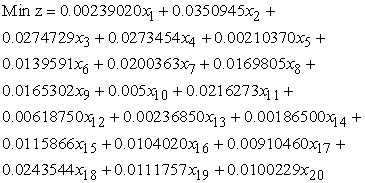In this problem, we will be looking for the values of X. The other values are provided as input. The problem constraints can then be written as follows. Coefficients are corresponding to 1 gram of the constraint and objective function

2.1.2. Constraints

Daily minimum standards of nutritional elements for Turkey (Paker, 1996) are given in the Table 3.

#### Table 3. Daily minimum standards of nutritional elements for Turkey

Our model is set up for breakfast in the morning, the weighted averages (thought to be three meals a day) 1/3 per cent constants were determined on the right-hand side. For example; energy’s the weighted average value is 2246 calorie for a day and we used it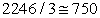for a breakfast.

We created constraints with the help of the Eq.2, Eq.3, Eq.4 and Eq.5 are as follows.

The minimum energy requirements for breakfast is set at 750 calorie per day.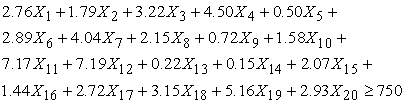The minimum protein requirements for breakfast is set at 23 gram per day.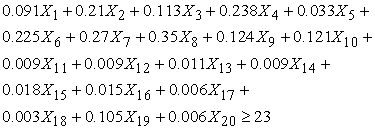The minimum oil requirements for breakfast is set at 24 gram per day.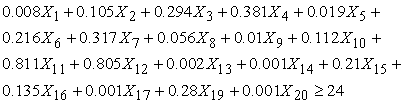The minimum carbohydrate requirements for breakfast is set at 109 gram per day.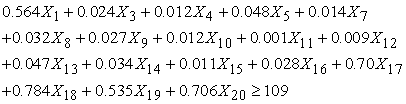The minimum calcium requirements for breakfast is set at 201 miligram per day.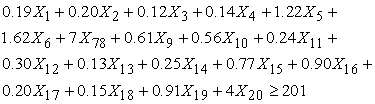The minimum iron requirements for breakfast is set at 5 miligram per day.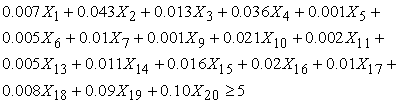The maximum potassium requirements for breakfast is set at 1167 miligram per day.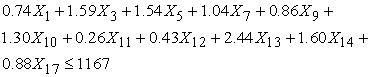The maximum sodium requirements for breakfast is set at 800 miligram per day.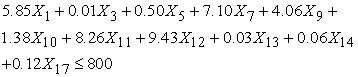The maximum Vitamin A requirements for breakfast is set at 1495 per day.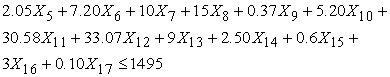The minimum Vitamin C requirements for breakfast is set at 21 per day.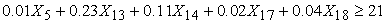and the other constraints as follows: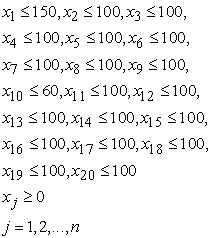The objective function is the value that we are trying to minimize. In our problem, the objective function is the cost of the entire foods. In our nutrition problem, the number of generations is fixed (up to 100), and the target is to provide effective and adequate nutrition.

2.2. Genetic Algorithm for Nutrition Problem

Genetic Algorithm is a random search algorithm that provides a robust method for searching for the optimum solution to complex problems (Goldberg,1989). In a GA, the problem is represented by a population of strings (or chromosomes, in biological terminology). Each string comprises a number of blocks, which represent the individual decision variables of the problem (genes). The variables represented in the string can be processed in an evaluation function or fitness function,which is in effect the objective function. Strings are processed and combined according to their fitness (objective function value) in order to generate new strings that contain the best features of two parent strings. Strings with the highest fitness have the greatest chance of contributing to future generations, as in the process of natural selection. Excellent introductions to GAs are given by (Goldberg,1989) and (Michalewicz ,1992).

Three fundamental operators are involved in manipulating strings and moving to a new generation: selection, crossover, and mutation. The approach taken to the operators of selection, crossover, and mutation can influence the results obtained, and different problems may require different approaches.

The main data structures in the Genetic Algorithm toolbox are chromosomes, objective function values and fitness values. The chromosome data structure stores an entire population in a single matrix of size Nind x Lind, where Nind is the number of individuals in the population and Lind is the length of the genotypic representation of those individuals. The decision variables in the genetic algorithm are obtained by applying some mapping from the chromosome representation into the decision variable space. An objective function is used to evaluate the performance of the decision variables in the problem domain. Fitness values are derived from objective function values through a scaling or ranking function.

The genetic algorithm uses three main types of rules at each step to create the next generation from the current population (Schmitt and Lothar, 2001), (Schmitt and Lothar, 2004):

•  Selection rules select the individuals, called parents, that contribute to the population at the next generation.

•  Crossover rules combine two parents to form children fort he next generation.

•  Mutation rules apply random changes to individual parents to form children.

In our nutrition problem, we used the Matlab – Genetic Algorithm toolbox for computational results of Genetic algorithm. We used “gatool” and minimize the our objective function (cost of the breakfast). We defined the objective function in a seperate m – file as shown in Figure 1.

2.3. Bayesian Optimization Algorithm for Nutrition Problem

This section discusses the proposed Bayesian optimization algorithm for the nutrition problem, including the construction of a Bayesian network.

2.3.1. The Construction of a Bayesian Network

Bayesian networks are also called directed graphical models, in which each node corresponds to one variable, and each variable corresponds to one position in the strings representing the solutions. The relationship between two variables is represented by a directed edge between the two corresponding nodes.

Bayesian networks are often used to model multinomial data with both discrete and continuous variables by encoding the relationship between the variables contained in the modeled data, which represents the structure of a problem. Furthermore, they are used to generate new data instances or variables instances with similar properties as those of given data. Figure 2 is the Bayesian network constructed for the nutrition problem, which is an acyclic directed graph representing the solution structure of the problem. Since a solution has no parents, it will be chosen from nodes according to their probabilities. The next will be chosen from nodes according to the probabilities conditioned on the previous nodes. This building process is repeated until the last node has been chosen. A path from nutrient 1 to nutrient m is thus formed where m is the number of the nutrient, representing a new potential solution. Since all probability values for each nutrient are normalized, we suggest the tournament method as a suitable strategy for rule selection (Goldberg, 1989).

The goal of learning is to find the variable values of all nodes that maximize the likelihood of the training data containing a number of independent cases. Thus, the learning in our case amounts to ‘counting’ based on a multinomial distribution. We used R programming for the Bayesian network. It calculates the conditional probabilities of each possible value for each node given all possible values of its parent nodes. Conditional probabilities as follows in Table 4.

#### Table 4. Conditional probabilities and value of probability

These probability values were used to generate new solutions. Then, the goal function with each decision variable being a probabilistic value corresponding to 1 gram of the objective coefficients were obtained by multiplying the price value.

2.3.2. A Bayesian Optimization Algorithm

This section introduces a Bayesian optimization algorithm for the nutrition problem and based on the estimation of conditional probabilities.

In the Bayesian optimization algorithm, the first population is generated at random. From the current population, a set of better strings is selected. Any selection method biased towards better fitness can be used, and in this paper, tournament selection is applied. The conditional probabilities of each node in the Bayesian network are computed. New strings are generated by using these conditional probability values, and are added into the population, replacing some of the old strings. The steps of the Bayesian optimization algorithm for nutrition problem are:

1. Set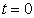, and generate an initial population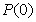at random;

2. Use tournament to select a set of promising strings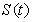from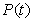;

3. Compute the conditional probabilities of each node according to this set of promising solutions;

4. For the each food, the tournament method is used to select string according to the conditional probabilities of all available nodes, thus obtaining a new string. A set of new strings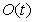will be generated in this way;

5. Create a new population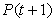by replacing some strings fromwith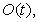and set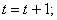6. If the termination conditions are not met (we use 100 generations), go to step 2.

### 3. Computational Results and Discussions

In this section we describe the computational experiments that were used to test BOA. For all experiments, real data sets as given to us by the TUIK are available. Data set consists of 20 nutrients and their prices. For all data instances, we used the following set of fixed parameters to implement our experiments. These parameters are based on our experience and intuition and thus are not necessarily the best for each instance. We have kept them the same for consistency.

3.1. Details of Algorithms

The detailed computational results over these 20 instances are listed in the tables.

•  LP: optimal solutions found with linear programming software (Dowsland and Thompson, 2000);

•  GA: optimal solutions found with genetic algorithm software (Matlab(R2009b))

•  BOA: optimal solutions found with Bayesian optimization algorithm

•  Stopping criterion: number of generations = 100, or an optimal solution is found

•  Population size = 100

•  The number of solutions kept in each generation = 20

•  Number of runs per data instance = 20

•  Creation Function = uniform

•  Selection Function = tournament

•  Crossover Function = two point

•  Hybrid Function = pattern search

The BOA was coded in Matlab (R2009b), and all experiments were run on the same Pentium (R) 1.60GHz PC with 1GB RAM using the Windows XP operating system. To test the robustness of the BOA, each data instance was run twenty times by fixing the above parameters and varying the pseudorandom number seed at the beginning.

3.2. Analysis of Results

First, let us see the results obtained from all of three methods.

The results obtained from the linear programming solution WinQSB package program: Bread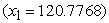, Milk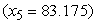, Egg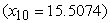, Margarine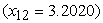, Tomato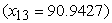, Sesame Oil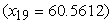and value of goal function (min.) is 1.4532.

The results obtained from the genetic algorithm solution: Bread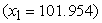, Wiener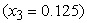., Salami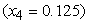, Milk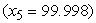, White Cheese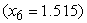, Cheddar Cheese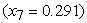, Curd Cheese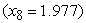, Egg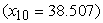, Buttter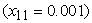, Margarine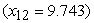, Tomato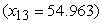, Cucumber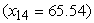, Black Olive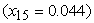, Green Olive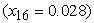, Jam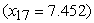, Sesame Oil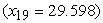, Boiled Grape Juice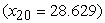and value of goal function (min.) is 1.7131.

The results obtained from the Bayesian optimization algorithm solution: Bread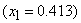, Sausage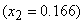, White Cheese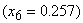, Cheddar Cheese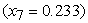, Curd Cheese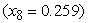, Egg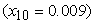, Margarine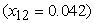, Jam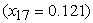, Honey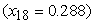, Sesame Oil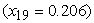, Boiled Grape Juice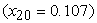and value of goal function (min.) is 1.017.

#### Table 5. All of the results obtained from Linear Programming, Genetic Algorithm and Bayesian Optimization Algorithm

Comparing the computational results on three methods, without learning the conditional probabilities, the results are much weaker. For the linear programming, 6 out of 20 foods are solved to optimality and this ratio for the genetic algorithm is 16 out of foods and for BOA is 11 out of 20 foods. Bayesian optimization algorithm solved the problem of nutrition in 11 seconds, genetic algorithm solved it in 15 seconds and linear programming solved it in 35 seconds. Thus, BOA has provided the solution to our problem in a shorter time than GA and LP. In terms of daily nutrient needs of the deviations, BOA had deviations lower than GA and LP. BOA solution is used to calculate the values of deviations of the posterior values, but the value of the deviation of each constraint is not possible to obtain from BOA. Therefore, only the total deviation value is written. The behavior of an individual run of the Bayesian algorithm is as expected. The optimal solution cost is 1.017 Turkish Lira. This is the least cost all of three methods. As a result, nutrient needs, and the cost advantage that deviations from the same to reduce the time to solve the problem in terms of nutrition for breakfast, Bayesian optimization algorithm can be used in an effective manner. All of the results are in the Table 5.

### 4. Conclusion

In this paper, BOA has been proposed to solve a nutrition problem. How to meet the daily needs of nutrients from food, at minimal cost and focusing on how much should be eaten. For this purpose, a linear programming model was created using real data, Matlab programming language software package, WinQSB packet program and R - Programming were analyzed. At the end of the comparisons, for the problem of a recommended diet for breakfast, BOA approach gives more effective results than the other mentioned methods in terms of time and the cost. BOA is an effective method to solve the problem about how to implement explicit learning from past solutions. Unlike most existing approaches, Bayesian Optimization Algorithm has the ability to build schedules by using flexible, rather than fixed rules. Experimental results from real-world nutrition problems have demonstrated the strength of the Bayesian optimization algorithm.

It is also hoped that this research will give some preliminary answers about how can we have a best price and nutritious breakfasts and may therefore be of interest to practitioners and researchers in areas of nutrition and evolutionary computation. In future, we will try to consider the problems of nutritional people or clinical examination of patients in special cases.

### Acknowledgement

The authors thank the anonymous referees for their interesting and helpful comments.

### References

  Alpaslan F. (1996), “Türkiye’de 6 Büyük İlde Doğrusal Programlama ile Optimum Beslenme Maliyetinin Minimizasyonu (1994-1997)”. Ondokuz Mayıs University, Fen-Edebiyat Fakültesi Araştırma Fonu. Yayın No: F.150.s. 6-8.In article  Anderson A.M. and Earle M.D. (1983), “Diet Planning in the Third World by Linear and Goal Programming”. J. Opl. Res. Soc. Vol.34. pp.9-16.In article CrossRef  Dantzig, G.B (1947), “Maximization of a linear function of variables subject to linear inequalities, T.C. Koopmans (ed.): Activity Analysis of Production and Allocation”, New York-London 1951 (Wiley & Chapman-Hall), pp. 339-347.In article  Dowsland KA, Thompson JM, (2000), Solving a nurse scheduling problem with knapsacks, networks and tabu search. J Oper Res Soc 51:825-833.In article CrossRef  Edwardson W. (1974), The Design of Nutritional Food Products for a Developing Country. A Thesis for the Degree of Ph. D. in Product Development, Massey University.In article  Kaldırım E. and Köse Z. (2006), “Application of a Multi-objective Genetic Algorithm to the Modified Diet Problem”, Genetic and Evolutionary Computation Congress (GECCO), Undergraduate Student Workshop, Seattle, USA.In article  Larranaga P., Lozano J.A., (2002), Estimation of Distribution Algorithms: A New Tool for Evolutionary Computation, Kluwer Academic Publishers, Boston.In article CrossRef  Lv Y. (2009), “Multi – Objective Nutritional Diet Optimization Based on Quantum Genetic Algorithm”; in Proc. ICNC (4), pp, 336-340.In article  Goldberg D.E. (1989), Genetic Algorithms in Search, Optimization and Machine Learning, Addison-Wesley, USA.In article  Michalewicz, Z. (1992). Genetic Algorithms + data Structures = evolution programs, Springer-Verlag, New York, Inc., New York.In article CrossRef  Muhlenbein H., Paaß G., (1996), “From recombination of genes to the estimation of distributions I. binary parameters”, PPSN IV: Proceedings of the 4th International Conference on Parallel Problem Solving from Nature, Springer-Verlag, pp. 178-187.In article  Paker H.S. (1996), Besinlerin Yenebilen 100 gramlarının Enerji ve Besin Öğeleri Değerleri, Sporda Beslenme, Gen Matbaacılık ve Reklamcılık, Ankara.In article  Pearl J., 1988. Probabilistic Reasoning in Intelligent Systems, Morgan Kaufman Publishers, Palo Alto, CA.In article  Pelikan M., Goldberg D.E., Cant´u-Paz E., (1999). BOA: The Bayesian optimization algorithm. Proceedings of the Genetic and Evolutionary Computation Conference (GECCO-99), I, 525-532. Also IlliGAL Report No. 99003.In article  Pelikan M., Goldberg D.E., (2000), “Hierarchical problem solving by the Bayesian optimization algorithm, in D. Whitley, D. Goldberg, E. Cant´u-Paz, L. Spector, I. Parmee & H.-G. Beyer (eds), Proceedings of the Genetic and Evolutionary Computation COnference (GECCO 2000), Morgan Kaufmann, pp. 267-274.In article  Sahingoz S.A. and Sanlier N., (2011), “Compliance with Mediterranean Diet Quality Index (KIDMED) and nutrition knowledge levels in adolescents. A case study from Turkey”, Appetite, Volume 57, Issue 1, August 2011, Pages 272-277.In article CrossRef  Schmitt, Lothar M.,(2001) “Theory of Genetic Algorithms”, Theoretical Computer Science, pp. 1-61.In article CrossRef  Schmitt, Lothar M., (2004) “Theory of Genetic Algorithms II: models for genetic operators over the string-tensor representation of populations and convergence to global optima for arbitrary fitness function under scaling”, Theoretical Computer Science, pp. 181-231.In article CrossRef  Sukhatme P.V., (1961), “The World's Hunger and Future Needs in Food Supplies”, Journal of Royal Statistical Society, Series A, Vol. 124, pp 463-525.In article CrossRef  TUIK (2012). Consumer Price Index. Available at: http://www.tuik.gov.tr. Accessed on 3 June 2013.In article